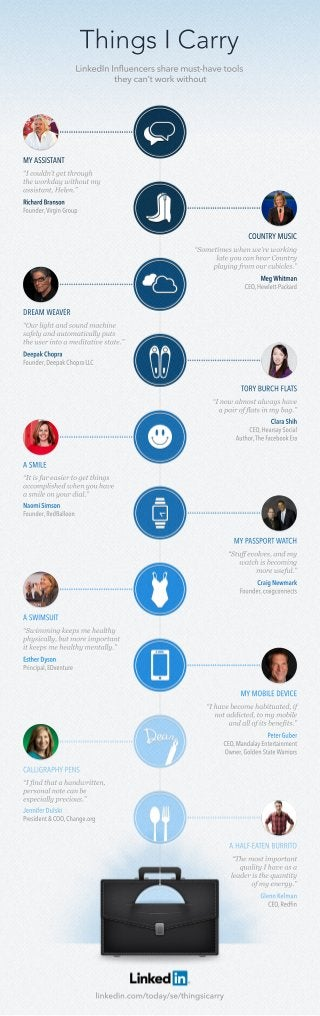Successfully reported this slideshow.×
1 of 1

Things I Carry: LinkedIn Influencers Infographic

62

Share

Wondering what secret weapon could take your productivity to the next level? We asked some of LinkedIn’s Influencers to describe their toolkits for success for “Things I Carry,” the latest in our series of special features by Influencers. Read more: http://www.linkedin.com/today/se/thingsicarry?trk=li_ss_0413

See all

See all

Things I Carry: LinkedIn Influencers Infographic

1. 1. Ti s C r h g I ar n y L k dnIf e c r saemut a etos i e I nl n es h r n u sh v o l - te c nt ok i o t h y a ’w r w t u h M AS TN Y SIA T S “cud ’ e tru h I o ln g tho g t te rd ywi o t h wok a t u my h as tn, l . si a tHe n” s e R hrBasn ia r o c d n Fudr iiGop one Vg r , rn u C U TY UI O NR M SC “o t s e we e rig S mei wh n ’ wokn me r lt y ucnh a C u ty ae o a e r o nr pa igf m o r u ie. lyn r o u cbc sl ” M g hm n eW i a t COH we- c r E,e lta a t kd P D EMW AE RA EVR “ u l h a dsu dmahn O ri t n o n g cie sfl a da tmai l p t ae n uo t al us y c y te srno me i t e tt. h ue it a dt i sae av ” D ea Cor epk hpa Fudr epk hp L one DeaCor L , aC TR B RHFAS O Y U C LT “n w amot l y h v I o l sawa s a e ap io f ti myb g” ar fl sn a a. C rSi la h a h COH a aScl E,er y oi s a At rh F eokr u o Tea bo E h, c a A ML SIE “tsa e s ro e tig Iifr ai t g thn s e acmpi e wh ny uh v co lh d e o a e s as lo y u da. mi n o r il e ” N o iisn am S o m Fudr eBlo one Rdaon , l M PSP R W TH Y ASO T AC “tfeovsa dmy Sufv le, n wac ibcmig th s eo n moe sfl r ueu. ” Ca N w a rg e m r i k Fudrrgonc one c i net ,a c s A WM U S ISIT “wi n ke s h aty S mmig ep me e l h p yi l ,u moemp ra t h s al b t r i otn c y ike s h atyme tl . t ep me e lh nal ” y Et r y n s eDs h o Pni lDet e rc aEvn r i p, u M M B E EI Y O I DV E L C “h v bcme a i ae ,f I a e eo h bt td i u n t d i e ,o mo i o a dc d t my bl t e a dalftbn fs n l i e ei . o s t” Pt G br e rue e COM na yn ri et E, adl Ettn n a ea m O nr o e S tW r r w e Gl n te ais , d a ro CLG AH PN AL RP Y ES I “f dta ah n wr tn Ii h t a d ie , n t p ro a n t cnb esn l oe a e ep c l peiu. sei l rc s ay o ” Jnir u k eneD l i f s P s et C OCag.g r i n& O ,hne r ed o A AF AE B RI H LET U R O - N T “h motmp ra t T e si otn q ai I a e s u ly h v a a t l d rsh q a ty e e ite u ni a t o mye eg . f n ry” Gen e a l Kl n n m CORdn E,ef i l k dnc m/ d ys/ ig i r i e i. n o t a /et n s ar o h c y